# Team:Aberdeen Scotland/Probability

University of Aberdeen - ayeSwitch - iGEM 2010

iGEM 2010

# Parameter Space Analysis

Recall that the equations of our system are as follows (see Equations page for parameter definitions):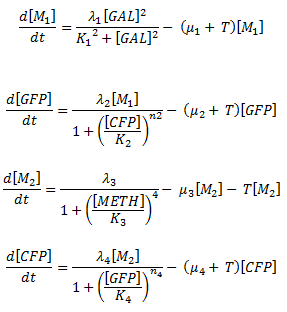We wrote a program which takes the equations for our system, calculates the nullclines and records whether or not bistability is achieved. Whether or not bistability is achieved depends strongly on the parameters λi, Ki, μi and ni.

The values for the parameters λi, Ki and μi were taken from literature- but have a large uncertainty attached to them. To take this uncertainty into account we selected each parameter value randomly from a range of two orders of magnitude around the literature value for each parameter. We knew that n2 was between 1 and 3 and n4=1. This is due to the number of stem loops on the two DNA strands (see Fig 1 in Equations). The strand associated with GFP has two stem loops. A protein can bind to one stem loop and this can then hinder the binding of a protein to the second stem loop (thus making n2 less than 2 but more than 1) or encourage the binding of a protein to the second stem loop (thus making n2 more than 2 but less than 3). The strand associated with the CFP has only one stem loop and thus n4=1. Please see Determination of the Hill coefficient n2 for an explanation on how we narrowed down the value for n2.

We ran the program for various combinations of Hill coefficients (ni) between 1 and 5. Each time the program was run, the Hill coefficients remained constant but the other parameters varied.

Choosing the parameters in this way meant that each time the program was run we would sometimes achieve a combination of parameters that allowed bistability, and sometimes not.

The program was run 100 times and the number of times bistability was achieved was output to the screen as a percentage.

We then decided to run the program for various different parameter ranges. We cannot change individual parameters specifically, but in the lab it is possible to vary the order of magnitude of a parameter. We wanted to know, if we varied our parameters and Hill coefficients, what is the best percentage we can possibly get? These results are shown in scenarios 2-4.

### Scenario 1 – unmodified parameters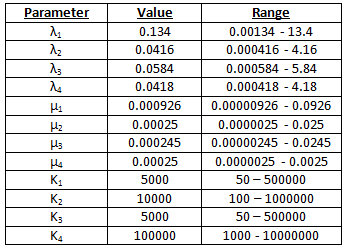These parameters were taken from, or derived from, the information contained within various sources of literature-. The parameters were converted from concentrations to numbers of molecules.

We did not know what concentration of galactose and methionine was optimal, so we ran various tests and found the value for which the percentages were maximised. We found that the optimal percentages occurred if we have 100000 times more galactose than methionine. See figure 1a and 1b.

a)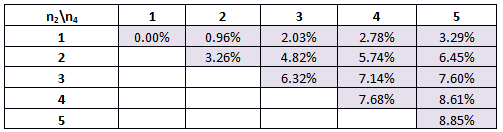b)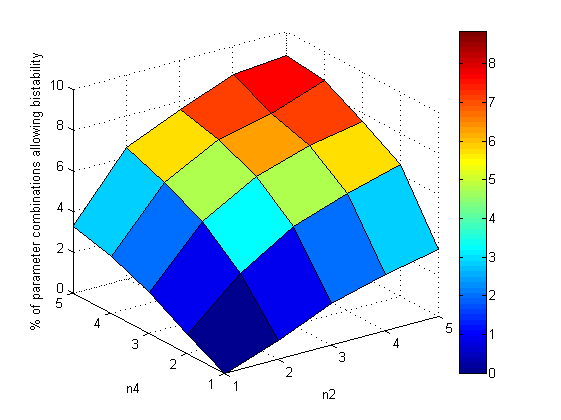Figure 1. a) The percentage of parameter combinations tested that will give us bistability for various combinations of Hill coefficients. n2 is the Hill coefficient for the GFP rate-reaction equation and n4 is the Hill coefficient for the CFP rate-reaction equation. b) Graphical representation of 1a.

Here we can see that if n4=1, then the best scenario we can hope for is that n2=5. In this situation, 3.29% of the parameter combinations tested will give bistability and the possibility of switching. However, with n2 estimated to be between 1 and 3, we can say that at most 2.03% of the parameter combinations tested gave bistability.

### Scenario 2 – modified parameters 1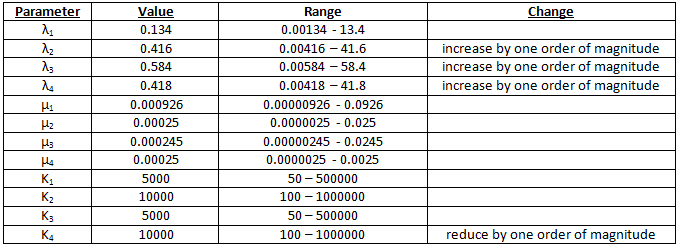Percentages are maximised if we have 100000 times more galactose than methionine. See figure 2a and 2b.

a)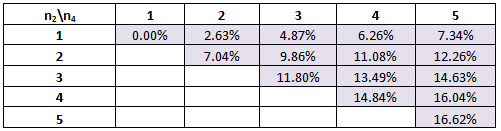b)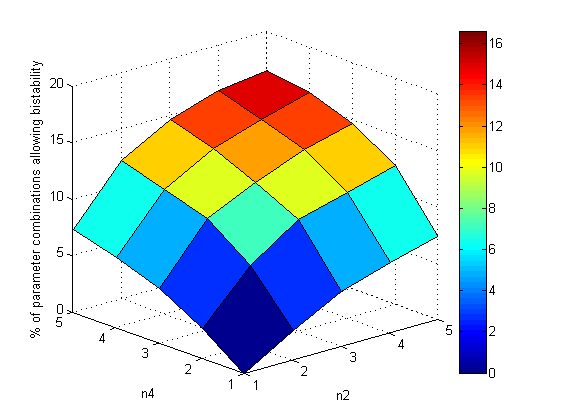Figure 2. a) The percentage of parameter combinations tested that will give us bistability for various combinations of Hill coefficients. b) Graphical representation of 2a.

The best scenario is if we have n2=5 and n4=5.

### Scenario 3 – modified parameters 2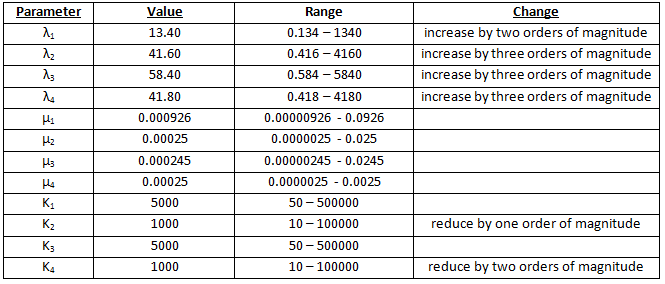Percentages are maximised if there is 100000 times more galactose than methionine. See figure 3a and 3b.

a)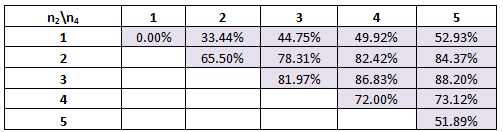b)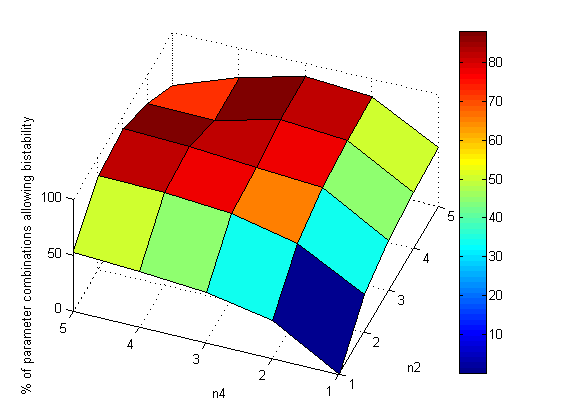Figure 3. a) The percentage of parameter combinations tested that will give us bistability for various combinations of Hill coefficients. b) Graphical representation of 3a.

Here we find that the optimal scenario is n2=3 and n4=5. The trend which has developed in this table will be discussed at the end of scenario 4.

### Scenario 4 – modified parameters 3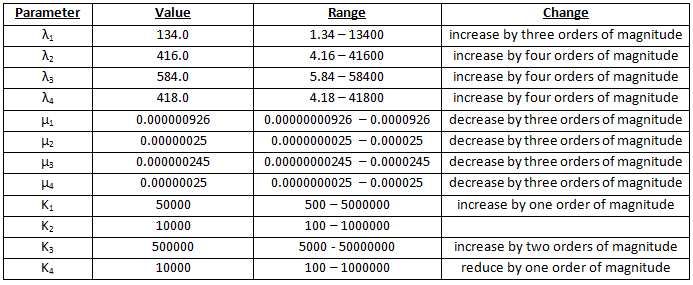Percentages are maximised if we have 10000000 times more galactose than methionine. See Figure 4a and 4b.

a)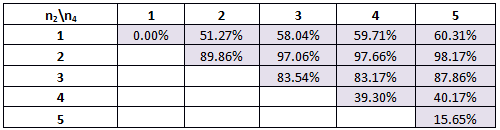b)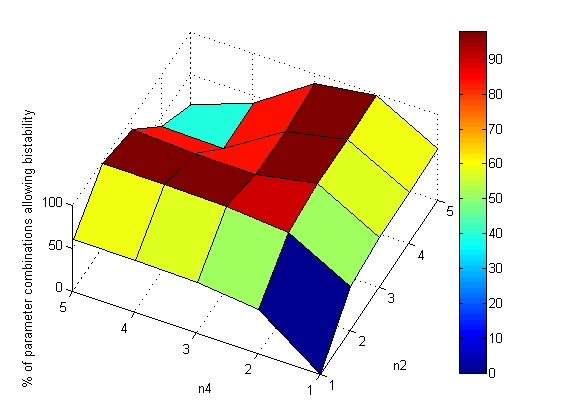Figure 4. a) The percentage of parameter combinations tested that will give us bistability for various combinations of Hill coefficients. b) Graphical representation of 4a.

An interesting trend is seen in scenarios 3 and 4. The percentages increase to a maximum at n2=2 and n4=5 (n2=3 and n4=5 in scenario 3) and then decrease, quite rapidly, towards Hill coefficients of n2=5 and n4=5. In the parameter space, there is a region where bistability is possible. For the smaller Hill coefficients, the randomly chosen parameters (as described above) would allow the nullclines to have a shape such that they would cross three or more times and bistability is achieved. The higher Hill coefficients, however, for the same range of parameters, would have nullclines which were shaped so that they did not cross three or more times – in parameter space, they fall outside the region of bistability.

### Conclusions

The ideal scenario is to change the parameters to that in scenario 4 and have Hill coefficients of n2=2 and n4=5. Here 98.17% of the parameter combinations tested gave bistability. Contrary to our initial assumptions, depending on the range of parameters, higher Hill coefficients are not always better. The parameters will dictate the shape and position of the nullclines and whether or not bistability is possible.

It is possible to determine the optimal values of the parameters for an ideal working of the switch. However, to change the parameters in the experimental construct so much would require much more time than we had available.

### References

 Hershey, J., Sonenberg, N., and Mathews, M. (2007), Origins and Principles of Translational Control. In Translational Control (pp.1-29). Cold Spring Harbor, NY: Cold Spring Harbor Press.

 Edwards, A.M., Kane, C.M., Young, R.A., and Kornberg, R.D. (1991), Two Dissociable Subunits of Yeast RNA Polymerase II Stimulate the Initiation of Transcription at a Promoter in Vitro, J. Biol. Chem., Vol. 266, No.1, pp.71-75

 Fürst, P., and Hamer, D. (1989), Cooperative activation of a eukaryotic transcription factor: Interaction between Cu(I) and yeast ACE1 protein, Proc. Natl. Acad. Sci. USA, Vol. 86, pp. 5267-5271

 Jensen, L.T., Posewitz, M.C., Srinivasan, C., and Winge, D.R. (1998), Mapping of the DNA binding domain of the copper-responsive transcription factor Mac1 from Saccharomyces cerevisiae, J. Biol. Chem., Vol. 273, No. 37, pp.23805-23811

 Rodgers, K.K., and Coleman, J.E. (1994), DNA binding and bending by the transcription factors GAL4(62*) and GAL4(149*), Protein Science, Vol.3, No. 4, pp. 608-619

 Garcia-Martinez, J., Aranda, A., and Perez-Ortin, J.E. (2004), Genomic run-on evaluates transcription rates for all yeast genes and identifies gene regulatory mechanisms, Mol. Cell., Vol. 15, No. 2, pp.303-313

 Wang, Y., Liu, C.L., Storey, J.D., Tibshirani, R.J., Herschlag, D., and Brown, P.O. (2002), Precision and functional specificity in mRNA decay, Proc. Natl. Acad. Sci. USA, Vol. 99, No.9, pp.5860-5865

 Beyer, A. et al. (2004), Post-transcriptional sxpression regulation in the Yeast Saccharomyces Cerevisiae on a genomic scale, Molecular and Cellular Proteomics, Vol. 3, No. 11, pp.1083-1092

 Mateus, C. and Avery, S.V. (2000), Destabilized green fluorescent protein for monitoring dynamic changes in yeast gene expression with flow cytometry, Yeast, Vol.16, No.14, pp.1313-1323

 Carey, J., Cameron, V., de Haseth, P.L., and Uhlenbeck, O.C. (1983), Sequence-specific interaction of R17 coat protein with its ribonucleic acid binding site, Biochemistry, Vol.22, No.11, pp.2601-2610

 Talbot, S.J., Goodman, S., Bates, S.R., Fishwick, C.W., and Stockley, P.G. (1990), Use of synthetic oligoribonucleotides to probe RNA-protein interactions in the MS2 translational operator complex, Nucleic Acids Res., Vol.18, No.12, pp.3521-3528

 Arava, Y., Wang, Y., Storey, J.D., Liu, C.L., Brown, P.O., and Herschlag, D. (2003), Genome-wide analysis of mRNA translation profiles in Saccharomyces cerevisiae, Proc. Natl. Acad. Sci. USA, Vol. 100, No.7, pp.3889-3894

 Chattopadhyay, S., Garcia-Mena, J., DeVito, J., Wolska, K., and Das, A. (1995), Bipartite function of a small RNA hairpin in transcription antitermination in bacteriophage lambda, Proc. Natl. Acad. Sci. USA, Vol.92, No.9, pp.4061-4065

Back to the Top